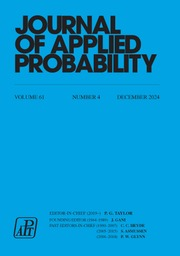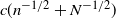Skip to main content Accessibility help
Home
Hostname: page-component-747cfc64b6-nq4kt Total loading time: 0.176 Render date: 2021-06-13T03:55:55.480Z Has data issue: true Feature Flags: { "shouldUseShareProductTool": true, "shouldUseHypothesis": true, "isUnsiloEnabled": true, "metricsAbstractViews": false, "figures": true, "newCiteModal": false, "newCitedByModal": true, "newEcommerce": true }Journal of Applied Probability

## Abstract

Motivated by mathematical tissue growth modelling, we consider the problem of approximating the dynamics of multicolor Pólya urn processes that start with large numbers of balls of different colors and run for a long time. Using strong approximation theorems for empirical and quantile processes, we establish Gaussian process approximations for the Pólya urn processes. The approximating processes are sums of a multivariate Brownian motion process and an independent linear drift with a random Gaussian coefficient. The dominating term between the two depends on the ratio of the number of time steps n to the initial number of balls N in the urn. We also establish an upper bound of the form$c(n^{-1/2}+N^{-1/2})$ for the maximum deviation over the class of convex Borel sets of the step-n urn composition distribution from the approximating normal law.

## MSC classification

Type
Research Papers
Information
Journal of Applied Probability , March 2021 , pp. 274 - 286
Copyright
© The Author(s), 2021. Published by Cambridge University Press on behalf of Applied Probability Trust

## Access options

Get access to the full version of this content by using one of the access options below.

## References

Athreya, K. B. and Ney, P. E. (1968). Embedding of urn schemes into continuous time Markov branching processes and related limit theorems. Ann. Math. Statist. 39, 18011817.CrossRefGoogle Scholar
Athreya, K. B. and Ney, P. E. (1972). Branching Processes. Springer, New York.CrossRefGoogle Scholar
Balakrishnan, N. and Rao, C. R. (1998). Order statistics: an introduction. In Handbook of Statistics, Vol. 16, Order Statistics: Theory and Methods, eds N. Balakrishnan and C. R. Rao. Elsevier Science, Amsterdam, pp. 3–24.CrossRefGoogle Scholar
Billingsley, P. (1999). Convergence of Probability Measures, 2nd edn. Wiley, New York.CrossRefGoogle Scholar
Binder, B. J., Landman, K. A. and Simpson, M. J. (2008) Modeling proliferative tissue growth: a general approach and an avian case study. Phis. Rev. E, 78, 031912.CrossRefGoogle Scholar
Binder, B. J. and Landman, K. A. (2009). Exclusion processes on a growing domain. J. Theoret. Biol. 259, 541551.CrossRefGoogle ScholarPubMed
Blackwell, D. and MacQueen, J. B. (1973). Ferguson distributions via Pólya urn schemes. Ann. Statist. 1, 353355.CrossRefGoogle Scholar
Blom, G. and Holst, L. (1991). Embedding procedures for discrete problems in probability. Math. Scientist, 16, 2940.Google Scholar
Cheliotis, D. and Kouloumpou, D. Functional limit theorems for the Pólya and q-Píya urns. Preprint, arXiv:1905.13336.Google Scholar
Csörgö, M. and Révész, P. (1978). Strong approximations of the quantile process. Ann. Statist. 6, 882894.CrossRefGoogle Scholar
Csörgö, M. and Révész, P. (1981). Strong Approximations in Probability and Statistics. Academic Press, New York.Google Scholar
de La Fortelle, A. (2006). Yule process sample path asymptotics. Electron. Commun. Prob. 11, 193199.CrossRefGoogle Scholar
Devroye, L., Mehrabian, A. and Reddad, T. (2018). The total variation distance between high-dimensional Gaussians. Preprint, arXiv:1810.08693.Google Scholar
Eggenberger, F. and Pólya, G. (1923). über die Statistik verketteter Vorgange. Z. Angew. Math. Mech. 3, 279289.CrossRefGoogle Scholar
Goldstein, L. and Reinert, G. (2013). Stein’s method for the Beta distribution and the Pólya–Eggenberger urn. J. App. Prob. 50, 11871205.CrossRefGoogle Scholar
Hewitt, E. and Savage, L. J. (1955). Symmetric measures on Cartesian products. Trans. Amer. Math. Soc. 80, 470501.CrossRefGoogle Scholar
Ikeda, S. and Matsunawa, T. (1972). On the uniform asymptotic normality of sample quantiles. Ann. Inst. Stat. Math. 24, 3352.CrossRefGoogle Scholar
Janson, S. (2004). Functional limit theorems for multitype branching processes and generalized Pólya urns. Stoch. Process Appl. 110, 177245.CrossRefGoogle Scholar
Janson, S. (2006). Limit theorems for triangular urn schemes. Prob. Theory Relat. Fields 134, 417452.CrossRefGoogle Scholar
Johnson, N. L. and Kotz, S. (1977). Urn Models and Their Applications. Springer, New York.Google Scholar
Komlos, J., Major, P. and Tusnady, G. (1975). An approximation of partial sums of independent RVs and the sample DF. I. Z. Wahrscheinlichkeitsth. 32, 111–131.CrossRefGoogle Scholar
Kotz, S. and Balakrishnan, N. (1997). Advances in urn models during the past two decades. In Advances in Combinatorial Methods and Applications to Probability and Statistics, ed. N. Balakrishnan. Birkhäuser, Boston, pp. 203–257.CrossRefGoogle Scholar
Mahmoud, H. M. (2009). Pólya Urn Models. CRC Press, Boca Raton.Google Scholar
Markov, A. A. (1906). Extension of the law of large numbers to quantities depending on each other. Izv. fizm.-mat. obsch. Kazanskom univ. 2, 135–156 (in Russian). [Reprinted in J. Electron. Hist. Prob. Statist. 2, Article 10 (2006).]Google Scholar
Matsunawa, T. (1975). On the error evaluation of the joint normal approximation for sample quantiles. Ann. Inst. Statist. Math. 27, 189199.CrossRefGoogle Scholar
Rachev, S. T., Klebanov, L. B., Stoyanov, S. V. and Fabozzi, F. (2013). The Methods of Distances in the Theory of Probability and Statistics. Springer, New York.CrossRefGoogle Scholar
Sazonov, V. V. (1975). On a bound for the rate of convergence in the multidimensional central limit theorem. In Proc. Sixth Berkeley Symp. on Math. Stat. and Prob., Berkeley and Los Angeles, University of California Press. Vol. 2, pp. 563–582.Google Scholar
Walker, A. M. (1968). A note on the asymptotic distribution of sample quantiles. J. R. Statist. Soc. B 30, 570575.Google Scholar

# Send article to Kindle

To send this article to your Kindle, first ensure no-reply@cambridge.org is added to your Approved Personal Document E-mail List under your Personal Document Settings on the Manage Your Content and Devices page of your Amazon account. Then enter the ‘name’ part of your Kindle email address below. Find out more about sending to your Kindle. Find out more about sending to your Kindle.

Note you can select to send to either the @free.kindle.com or @kindle.com variations. ‘@free.kindle.com’ emails are free but can only be sent to your device when it is connected to wi-fi. ‘@kindle.com’ emails can be delivered even when you are not connected to wi-fi, but note that service fees apply.

Find out more about the Kindle Personal Document Service.

Gaussian process approximations for multicolor Pólya urn models
Available formats
×

# Send article to Dropbox

To send this article to your Dropbox account, please select one or more formats and confirm that you agree to abide by our usage policies. If this is the first time you use this feature, you will be asked to authorise Cambridge Core to connect with your <service> account. Find out more about sending content to Dropbox.

Gaussian process approximations for multicolor Pólya urn models
Available formats
×

# Send article to Google Drive

To send this article to your Google Drive account, please select one or more formats and confirm that you agree to abide by our usage policies. If this is the first time you use this feature, you will be asked to authorise Cambridge Core to connect with your <service> account. Find out more about sending content to Google Drive.

Gaussian process approximations for multicolor Pólya urn models
Available formats
×
×

#### Reply to:Submit a response

Please enter your response.

#### Your details

Please enter a valid email address.

#### Conflicting interests

Do you have any conflicting interests? *